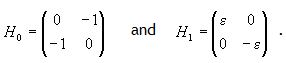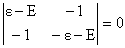### Mixed method problems

#### Problem:

Consider the 1D infinite well, U(x) = 0 for 0 < x < L, U(x) infinite everywhere else.
The eigenfunctions and eigenvalues of the Hamiltonian for a particle of mass m trapped in this well are
ψn(x) = (2/L)½sin(nπx/L), En = n2π2ħ2/(2mL2), respectively.
Now consider the perturbed 1D infinite well, U(x) = 0 for 0 < x < L/2, U(x) = U0 for L/2 < x < L, U(x) infinite everywhere else.
Let E0 denote the ground state energy of the unperturbed well.
Assume U0 = E0/10 = 0.1*π2ħ2/(2mL2),  i.e. U0 = 0.1 times the ground state energy of the unperturbed well.

(a)  Solve for the energy eigenvalues of the perturbed well without using an approximation method.
(b)  Solve for the energy eigenvalues of the perturbed well using the WKB approximation.
(c)  Solve for the energy eigenvalues of the perturbed well using 1st order perturbation theory.
(c)  Solve for the lowest energy eigenvalues of the perturbed well using the variational method.

Solution:

• Concepts:
Comparing approximation methods
• Reasoning:
We are asked to solve for the energy eigenvalues of the perturbed well using various approximation methods.
• Details of the calculation:
(a)  No approximation:
We have a piecewise constant potential.
Let 0 < x < L/2 define region 1 and L/2 < x < L define region 2.
The most general solution of the time independent solution in region 1 satisfying the boundary condition at x = 0 is
ψ1(x) = A sin(k1x)
The most general solution of the time independent solution in region 2 satisfying the boundary condition at x = L is
ψ2(x) = B sin(k2(L-x)).
Here k12 = (2m/ħ2)E and k22 = (2m/ħ2)(E - U0).
The boundary conditions are that ψ(x) and (∂/∂x)ψ(x) are continuous at x = L/2.
A sin(k1L/2) = B sin(k2L/2).
k1A cos(k1L/2) = -k2B cos(k2L/2).
This yields tan(k1L/2)/(k1L/2) = -tan(k2L/2)/(k2L/2).
This equation can be solved numerically.  We expect solutions for values of k1L/2 = nπ/2 + ε1 and k2L/2 = nπ/2 - ε2, where ε1 and ε2 are small positive numbers.
Using Excel to find the ground state energy of the perturbed well E1 we obtain E1 = 1.04936 E0
E1 = E0 + 0.4936 U0.

(b)  The WKB approximation:
In one dimension, if U(x) finite everywhere, then the WKB approximation requires
cylcepdx = ∫cylceħkdx = (n - ½)h n = 1, 2, ... .
Here U(x) is infinite everywhere except in some finite region and ∫cylcepdx = ∫cylceħkdx = nh.
0L ħkdx = nh/2 = nπħ.  ∫0L/2 (2mE)½dx + ∫L/2L (2m(E-U0))½dx = nπħ.
(2mE)½L/2 + (2m(E - U0))½L/2 = nπħ.
E/4 + (E - U0)/4 + (E(E - U0))½/2 = n2π2ħ2/(2mL2) = n2E0.
E/2 - U0/4 + (E/2)(1 - U0/E)½ ~ E/2 - U0/4 + E/2 - U0/4 = E - U0/2 = n2E0.
E = n2E0 + U0/2, keeping only first order terms in the expansion.
Higher precision solution of E/2 - U0/4 + (E/2)(1 - U0/E)½ = E0 we obtain
E1 = E0 + 0.506 U0.

(c)  First order perturbation theory:
En = n2π2ħ2/(2mL2) + (2/L)∫L/2L sin2(πx/l)U0)dx =  n2π2ħ2/(2mL2) + 0.5 U0.
E1 = E0 +  0.5 U0.

(d) The variational method:
We have to choose a trial wave function with an adjustable parameter which satisfies the boundary conditions.
Choose  ψ(x) = A (2/L)½(sin(πx/L) + bsin(2πx/L)) = A(ψ1(x) + bψ2(x)).
Normalize: A2(1 + b2) = 1,  A = (1/(1 + b2))½.
<H> = <ψ|H|ψ> = -(ħ2/(2m))∫0L ψ*(∂2/∂x2)ψ dx + U0L/2L ψ*ψ dx.
<H> = A2E1(unperturbed) + A2b2E2(unperturbed) + U0[A2L/2Lψ11 dx + A2b2L/2Lψ22 dx + 2A2b(2/L)∫L/2Lsin(πx/L) sin(2πx/L) dx]
= E0[(1 + 4b2)/(1 + b2) + 0.1*[0.5(1 + b2)/(1 + b2) - (8/(3π))b/(1 + b2)]
= E0[(1 + 4b2)/(1 + b2) + 0.05 - (8/(30π))b/(1 + b2)].
d<H>/db = 0.  6b - (8/(30π))(1 - b2) = 0.  b2 + 22.5π b - 1 = 0.
b = -11.25 π + ((11.25 π)2 + 1)½ = +0.01414
<H>min = E0*1.0494
E1 = E0 + 0.494 U0 is the best estimate for the ground state energy using the variational method.#### Problem:

Let(a)  Find the eigenvalues and eigenvectors of H0.
(b)  Now derive the expressions for E(1), the change in energy to first order in H1.  Also derive an equation which must be satisfied for the first order correction |ψ>(1).  Use these results to find E(1) and |ψ>(1) for each of the unperturbed states.
(c)  Now solve (H0 + H1)|ψ> = E|ψ> exactly, obtaining both eigenvalues and eigenvectors.  Expand your results to first order in ε and verify that they agree with the results from part (b).  [Note: Your exact answers for the eigenvectors should be normalized.]

Solution:

• Concepts:
Two-state problem, first-order perturbation theory
• Reasoning:
We are asked to find the exact eigenvalues of a Hamiltonian operator in a two-dimensional vector space and to find its approximate eigenvalues to first order.
• Details of the calculation:
(a)  We diagonalize the matrix of H0..

E2 = 1, E± = ±1.  |ψ+> = 2(|1> - |2>),  |ψ-> = 2(|1> + |2>).

(b)  For the two state system we have
H010> = E0110> = 1*|ψ+>,  H020> = E0220> = -1*|ψ->.
Let H = H0 + λW.  Let |ψ1> and |ψ2> be the eigenstates of H.
H|ψ1> = E11>,  H|ψ2> = E22>.
Assume λW = H1, and λ is small.
Since λW is small, we assume that E and |ψ> can be expanded as a power series in λ.
Ep = E0p + λE1p + λ2E2p + ...  ,
p> = |ψp0> + λ|ψp1> + λ2p2> + ...  .
(For the two-state system p can only take on the values 1 or 2.)
We may then write
(H0 + λW)(|ψp0> + λ|ψp1> + λ2p2> + ...)
= (E0p + λE1p + λ2E2p + ...)(|ψp0> + λ|ψp1> + λ2p2> + ...).
This equation is must be valid over a continuous range of λ.  Therefore we equate coefficients of equal powers of λ on both sides to obtain a series of equations that represent successively higher orders of the perturbation.
(i)  (H0 - E0p)|ψp0> = 0
(ii)  (H0 - E0p)|ψp1> = (E1p - W)|ψp0>
(iii)  (H0 - E0p)|ψp2> = (E1p - W)|ψp1> + E2pp0>
We choose <ψp0p0> = 1.  |ψps> is not uniquely defined.  We can add an arbitrary multiple of |ψp0> to each |ψps> without affecting the left hand side of the above equations.  Most often this multiple is chosen so that <ψp0ps> = 0.  The perturbed ket is then not normalized.
Multiplying (ii) by <ψp0| (and then setting λ = 1) we have  E1p = <ψp0|H1p0>.
E1p is the first order energy correction.
p1> may be written as |ψp1> = ∑p'bp'p'0>.  Here bp = 0 since <ψp0p1> = 0.
For our two-state system we therefore can write
11> = b220>, |ψ21> = b110>.
Using (ii) we write
(H0 - E01)|ψ11> = (E11 - H1)|ψ10>,
b2(H0 - E01)|ψ20> = b2(E02 - E01)|ψ20> = (E11 - H1)|ψ10>.
Multiplying by <ψ20| we obtain
b2 = <ψ20|H110>/(E01 - E02).
Similarly,
b1 = <ψ10|H120>/(E02 - E01).
In this problem
+|H1+> = ½[<1|H1|1> + <2|H1|2> - <1|H1|2> - <2|H1|1>) = 0.
-|H1-> = ½[<1|H1|1> + <2|H1|2> + <1|H1|2> + <2|H1|1>) = 0.
+|H1-> = ½[<1|H1|1> - <2|H1|2> + <1|H1|2> - <2|H1|1>) = ε.
-|H1+> = ½[<1|H1|1> - <2|H1|2> - <1|H1|2> + <2|H1|1>) = ε.
Therefore E11 = E12 = 0, the first order energy corrections are zero.
E1 = E+ and E2 = E- to first order.
To first order the eigenstates of H are
1> = |ψ+> + (ε/2)|ψ->,  |ψ2> = |ψ-> - (ε/2)|ψ+>.
1> = 2[(1 + (ε/2))|1> - (1 - (ε/2))|2>],
2> = 2[(1 - (ε/2))|1> + (1 + (ε/2))|2>].

(c)  We diagonalize the matrix of H..

(E - ε)(E + ε) = 1,  E2 = 1 + ε2,  E = ±(1 + ε2)½ ~ ±(1 + ε2/2).
E1 = E+ = 1 and E2 = E- = -1 to first order in ε.
1> = a|1> + b|2>, where a(ε - (1 + ε2)½) - b = 0,  a2 + b2 = 1.
2> = c|1> + d|2>, where c(ε + (1 + ε2)½) - d = 0,  c2 + d2 = 1.
Expand and keep only terms up to first order in ε.
a(ε - 1) - b = 0, a2 + a2(ε - 1)2 = 1,  a2 + a2(1 - 2ε) = 1,
a2 = 1/(2 - 2ε), b2 = (1 - 2ε)/(2 - 2ε) ~ (1 - ε)/2
a ~ 2((1 + (ε/2)),  b ~ -2((1 - (ε/2)).
c(ε + 1) - d = 0, c2 + c2(ε + 1)2 = 1,  c2 + c2(1 + 2ε) = 1,
c2 = 1/(2 + 2ε), d2 = (1 + 2ε)/(2 + 2ε) ~( 1 + ε)/2.
c ~ 2((1 - (ε/2)),  d ~ 2((1 + (ε/2)).
If we keep only terms up to first order in ε we get the same solution as in part b.